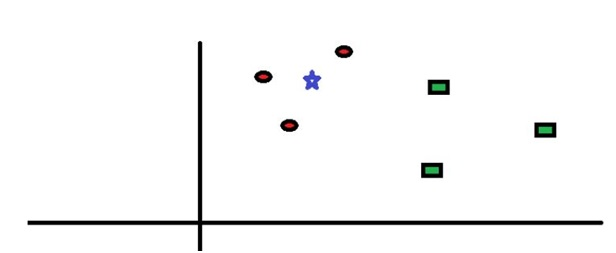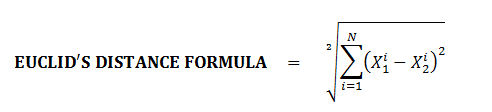# K-Nearest Neighbor (KNN) Algorithm and Its Implementation using Python

In this tutorial, we will learn about the K-Nearest Neighbor (KNN) algorithm and Its implementation using Python. By Ritik Aggarwal Last updated : April 16, 2023

Goal: To classify a query point (with 2 features) using training data of 2 classes using KNN.

## What is K-Nearest Neighbor (KNN) Algorithm?

The K-Nearest Neighbor (KNN) is a basic machine learning algorithm that can be used for both classifications as well as regression problems but has limited uses as a regression problem. So, we would discuss classification problems only.

It involves finding the distance of a query point with the training points in the training datasets. Sorting the distances and picking k points with the least distance. Then check which class these k points belong to and the class with maximum appearance is the predicted class.Red and green are two classes here, and we have to predict the class of star point. So, from the image, it is clear that the points of the red class are much closer than points of green class so the class prediction will be red for this point.We will generally work on the matrix, and make use of "numpy" libraries to evaluate this Euclid’s distance.

### Steps for algorithm

• STEP 1: Take the distance of a query point or a query reading from all the training points in the training dataset.
• STEP 2: Sort the distance in increasing order and pick the k points with the least distance.
• STEP 3: Check the majority of class in these k points.
• STEP 4: Class with the maximum majority is the predicted class of the point.

Note: In the code, we have taken only two features for a better explanation but the code works for N features also just you have to generate training data of n features and a query point of n features. Further, I have used numpy to generate two feature data.

## Python Code to Implement K-Nearest Neighbor (KNN) Algorithm

```import numpy as np

def distance(v1, v2):
# Eucledian
return np.sqrt(((v1-v2)**2).sum())

def knn(train, test, k=5):
dist = []

for i in range(train.shape):
# Get the vector and label
ix = train[i, :-1]
iy = train[i, -1]
# Compute the distance from test point
d = distance(test, ix)
dist.append([d, iy])
# Sort based on distance and get top k
dk = sorted(dist, key=lambda x: x)[:k]
# Retrieve only the labels
labels = np.array(dk)[:, -1]

# Get frequencies of each label
output = np.unique(labels, return_counts=True)
# Find max frequency and corresponding label
index = np.argmax(output)
return output[index]

# monkey_data && chimp data
# Data has 2 features
monkey_data = np.random.multivariate_normal([1.0,2.0],[[1.5,0.5],[0.5,1]],1000)
chimp_data = np.random.multivariate_normal([4.0,4.0],[[1,0],[0,1.8]],1000)

data = np.zeros((2000,3))
data[:1000,:-1] = monkey_data
data[1000:,:-1] = chimp_data
data[1000:,-1] = 1

label_to_class = {1:'chimp', 0 : 'monkey'}

## query point for the check
print("Enter the 1st feature")
x = input()
print("Enter the 2nd feature")
y = input()

x = float(x)
y = float(y)

query = np.array([x,y])
ans = knn(data, query)

print("the predicted class for the points is {}".format(label_to_class[ans]))
```

### Output

```Enter the 1st feature
3
Enter the 2nd feature
2
the predicted class for the points is chimp
```